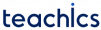# Stack

A stack is a linear data structure in which an element may be inserted or deleted only at one end, called the top of the stack. That is elements are removed from a stack in the reverse order of insertion. Thus stacks are also called LIFO(Last In First Out) lists. The following figure shows a real-life example of such a structure: a stack of boxes.

Here you can

• Put a new box on the top
• Remove the top box

To remove the box at the bottom, you should remove all boxes on the top.

## Basic Operations on Stack

There are three basic operations on a stack.

• PUSH – Inserting an element into a stack.
• POP – Deleting an element from a stack.
• PEEK – Returns the topmost element of the stack.

Both PUSH, POP and PEEK operations are performed on the top of the stack.

### PUSH Operation on a Stack

Inserting a new element in the TOP of the stack is called the PUSH operation. We must check if the stack is full before insertion. After PUSH operation the TOP will point to the newly inserted item.

In the following figure, item 7 will be added into the stack on the top of item 6 and after insertion, the TOP pointer will point to item 7.

#### Stack Overflow Condition

In computer programs, the size of the stack is predefined. If we try to insert a new element into a full stack, then the stack overflow condition will occur.

### POP Operation on a Stack

The POP operation deletes an element from the top of the stack. We must check if the stack is empty before deletion. The value of TOP will be decremented by one after the POP operation. In the figure, item 6 will be removed from the stack and now the TOP will be pointing to item 5.

#### Stack Underflow Condition

Stack underflow condition occurs when we try to remove an item from an empty stack.

### PEEK Operation on a Stack

Peek is an operation that returns the value of the topmost element of the stack. This operation doesn’t delete the element.

Here the PEEK operation will return the value 7 without deleting it.

## Representation of Stacks

Stack is usually represented by means of a linear array or a one-way linked list.

### Array Representation of Stacks

Stacks can be maintained in a program by using a linear array of elements and pointer variables TOP and MAX. The TOP pointer contains the location of the top element of the stack and MAX gives the maximum number of elements in the stack.

The stack is empty if TOP=-1 or TOP=NULL. The following figure shows an array representation of the stack.

TOP=3 indicates that the stack has four elements(0 to 3). Since MAX-1=9, there is space for 6 more elements in the stack.

The PUSH and POP operations can be implemented in a stack by the following algorithms.

#### PUSH(STACK, TOP, MAX, ITEM)

```1. If TOP=MAX-1, then Print: OVERFLOW, and Return
2. Set TOP=TOP+1
3. Set STACK[TOP]=ITEM
4. Return ```

#### POP(STACK, TOP, ITEM)

```1. If TOP=-1, then Print: UNDERFLOW, and Return
2. Set ITEM=STACK[TOP]
3. Set TOP=TOP-1
4. Return ```

#### PEEK(STACK, TOP)

```1. If TOP=-1, then Print: UNDERFLOW, and Return
2. RETURN STACK[TOP]```

These three operations can be implemented in C as follows

```void push()
{
if (top == MAX - 1)
{
printf("\n STACK OVERFLOW");
}
else
{
top++;
st[top] = val;
}
}
int pop()
{
int val;
if (top == -1)
{
printf("\n STACK UNDERFLOW");
return -1;
}
else
{
val = st[top];
top--;
return val;
}
}
void display()
{
int i;
if (top == -1)
printf("\n STACK IS EMPTY");
else
{
for (i = top; i >= 0; i--)
printf("\n %d", st[i]);
}
}
int peek()
{
if (top == -1)
{
printf("\n STACK IS EMPTY");
return -1;
}
else
return (st[top]);
}```

The disadvantage of using an array to represent a stack is that the array must be specified to have a fixed size. If the size of the array can’t be determined in advance, we can use the linked representation of the stack. The HEAD pointer of the linked list is used as the TOP of the stack.

The PUSH and POP operations can be implemented in a stack by the following algorithms.

```1. Allocate memory for the new node and name it as NEW
2. Set NEW->DATA=ITEM
3. IF TOP=NULL
SET NEW->NEXT=NULL
SET TOP=NEW
ELSE
SET NEW->NEXT-TOP
Set TOP=NEW
[END OF IF]
6. Exit.```

The operation is the same as inserting a new node at the beginning of a linked list.

```1. IF TOP=NULL then Write UNDERFLOW and Exit
2. Set ITEM=STACK->DATA
4. FREE TEMP
5. Exit```

The operation is the same as deleting a node from the beginning of a linked list.